Thom catastrophes

(diff) ← Older revision | Latest revision (diff) | Newer revision → (diff)

Singularities of differentiable mappings, whose classification was announced by R. Thom  in terms of their gradient dynamical systems and the analogous list of critical points of codimension(cf. Critical point) of differentiable functions. The original formulation of Thom's result is that a generic four-parameter family of functions is stable, and in the neighbourhood of a critical point it behaves, up to sign and change of variable, like one of seven cases (cf. Table).

<tbody> </tbody>
 Notation Codim Corank Germ Universal deformation Name1 1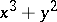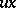Fold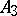2 1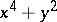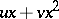Cusp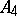3 1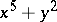Swallow-tail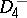3 2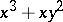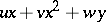Hyperbolic umbilic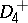3 2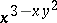Elliptic umbilic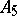4 2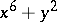Butterfly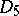4 2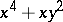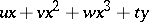Parabolic umbilic

The germs (cf. Germ) corresponding to the Thom catastrophes are finitely determined (specifically,-determined: in appropriate coordinates they correspond to polynomials in two variables of degrees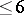).

The codimension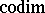serves as a measure of the complexity of a critical point. Any small perturbation of a functionof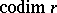leads to a function with at mostcomplex critical points. The codimension of a singularity (that is, of a germsuch that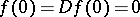) is the number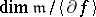, whereand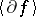is the ideal generated by the germs. For example, if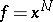, then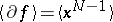, and the residue classes of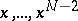form a basis of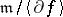, so that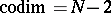. The inequality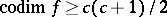, holds, whereis the corank of the Hessian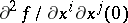. Hence, in particular, if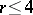, then.

Finite determination (sufficiency) of a germ, roughly speaking, means that it is determined, up to smooth change of coordinates, by its jets (cf. Jet). More precisely, a germis said to be-determined if every germ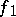with the same-jet (that is, the same Taylor series up to order) asis right equivalent to(i.e.where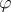is the germ atof a diffeomorphism; cf. ). A germ is finitely determined if and only if it has finite codimension. In particular, if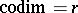, thenis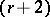-determined (whence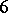-determined for).

The Thom catastrophes, in contrast to the case of general position, are degenerate singularities (that is, the Hessian is degenerate at them), and they can be removed by a small perturbation, as mentioned above. However, in many cases of practical importance, and also theoretically, one is interested not in an individual object, but in a collection of them, depending on some "control" parameters. Degenerate singularities which are removable for each fixed value of the parameters may be removable for the collection as a whole. (Stability of Thom catastrophes may also be considered in this sense.) But then the natural object of study is not the singularity itself, but a collection (a deformation of the singularity) in which it is non-removable (or disintegrates, or "bifurcates" ) under a change of parameters. It turns out that in many cases the study of all possible deformations can be reduced to the study of a single one, which is in a certain sense so big that all the others can be obtained from it. Such deformations are called versal and they, in turn, can be obtained from a universal (or miniversal) deformation, which is characterized by having least possible dimension of its parameter space. The most important result here is Mather's theorem: A singularityhas a universal deformation if and only if its codimension is finite.

A deformation,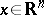,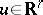, of a germ,, is given by a formula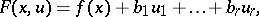where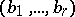is an arbitrary collection of representative elements of a basis of the space. Thom catastrophes correspond to deformations with at most four parameters.

Important for applications is the so-called bifurcation set, or singular set,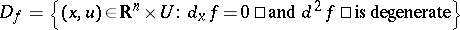; its projection to the-space, the set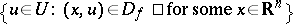, is called the catastrophe set. It lies in the control space and hence is "observable" , and all "discontinuities" or "catastrophes" originate from it. Fig.1a, Fig.1b and Fig.1c illustrate the cases corresponding to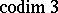.Figure: t092650a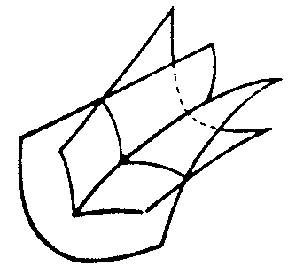Figure: t092650b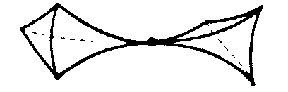Figure: t092650c